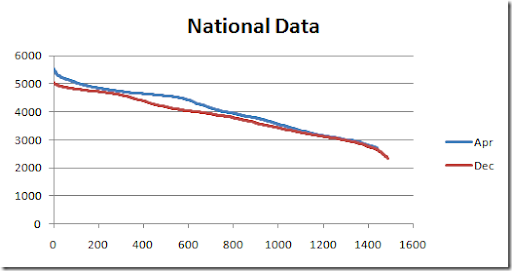## Wednesday, March 31, 2010

### Creating Load Duration Curves with GAMS

In power generation analysts often want to see a Load Duration Curve (http://en.wikipedia.org/wiki/Load_duration_curve). This involves sorting, a task that is not always immediately obvious how to do in GAMS. Here is some optimized code to perform the creation of a load curve:

alias (st,month,m);
alias (d,day);
set k /k1*k10000/;

dt(d,tp)\$

* map (d,tp) onto a 1 dim set k.
option map(dt:k);

set monthsub(month) 'only months that are really used';
monthsub(m)\$(

* gdxrank only sorts one dimensional parameters so we use mapping
parameter unsorted(k),sorted(k);
parameter indx(k);
\$libinclude rank
loop(monthsub(m),
unsorted(k) =
\$  libinclude rank unsorted k indx
sorted(k + (
card(k) + 1 - indx(k) - ord(k)) ) = unsorted(k);